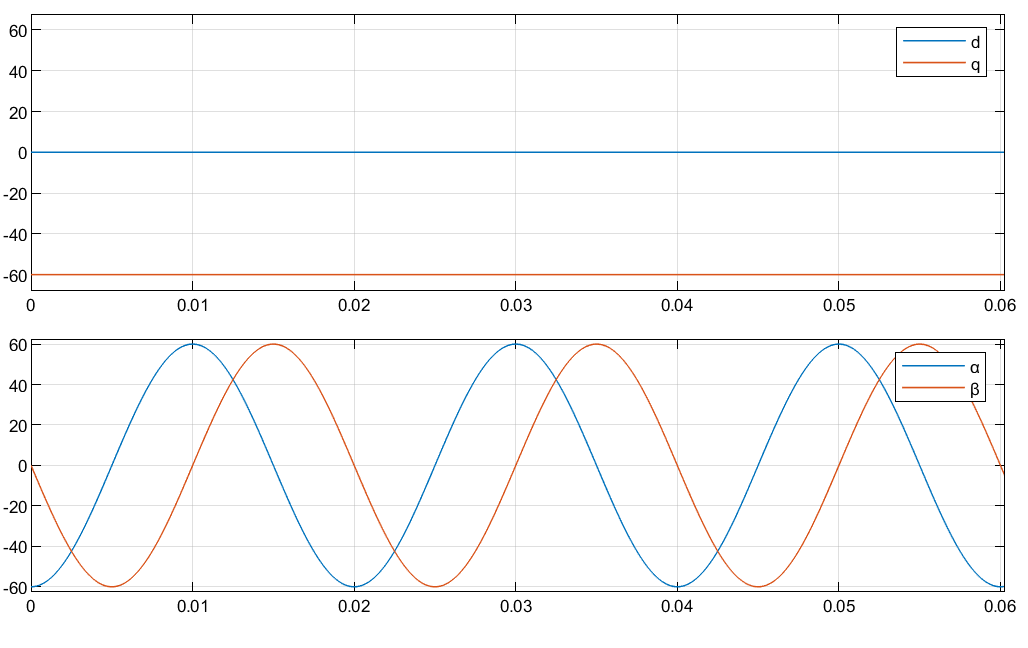Inverse Park Transform

Implement dq to αβ transformation

• Library:
• Motor Control Blockset / Controls / Math Transforms

•Description

The Inverse Park Transform block computes the inverse Park transformation of the orthogonal direct and quadrature axes components in the rotating dq reference frame. You can configure the block to align either the d- or q-axis with the α-axis at time t = 0.

The block accepts the following inputs:

• d-q axes components in the rotating reference frame.

• Sine and cosine values of the corresponding angles of transformation.

It outputs the two-phase orthogonal components in the stationary αβ reference frame.

The figures show a rotating dq reference frame and the α-β axes components in an αβ reference frame for when:

• The d-axis aligns with the α-axis.• The q-axis aligns with the α-axis.In both cases, the angle θ = ωt, where:

• θ is the angle between the α- and d-axes for the d-axis alignment or the angle between the α- and q-axes for the q-axis alignment. It indicates the angular position of the rotating dq reference frame with respect to the α-axis.

• ω is the rotational speed of the d-q reference frame.

• t is the time, in seconds, from the initial alignment.

The figures show the time-response of the individual components of the αβ and dq reference frames when:

• The d-axis aligns with the α-axis.• The q-axis aligns with the α-axis.Equations

The following equations describe how the block implements inverse Park transformation.

• When the d-axis aligns with the α-axis.

• When the q-axis aligns with the α-axis.

where:

• ${f}_{d}$ and ${f}_{q}$ are the direct and quadrature axis orthogonal components in the rotating dq reference frame.

• ${f}_{\alpha }$ and ${f}_{\beta }$ are the two-phase orthogonal components in the stationary αβ reference frame.

Ports

Input

expand all

Direct axis component, d, in the rotating dq reference frame.

Data Types: single | double | fixed point

Quadrature axis component, q, in the rotating dq reference frame.

Data Types: single | double | fixed point

Sine value of the angle of transformation, θe. θe is the angle between the rotating reference frame and the α-axis.

Data Types: single | double | fixed point

Cosine value of the angle of transformation, θe. θe is the angle between the rotating reference frame and the α-axis.

Data Types: single | double | fixed point

Output

expand all

Alpha-axis component, α, in the stationary αβ reference frame.

Data Types: single | double | fixed point

Beta-axis component, β, in the stationary αβ reference frame.

Data Types: single | double | fixed point

Parameters

expand all

Align either the d- or q-axis of the rotating reference frame to the α-axis of the stationary reference frame.

Extended Capabilities

Fixed-Point ConversionDesign and simulate fixed-point systems using Fixed-Point Designer™.

Introduced in R2020a